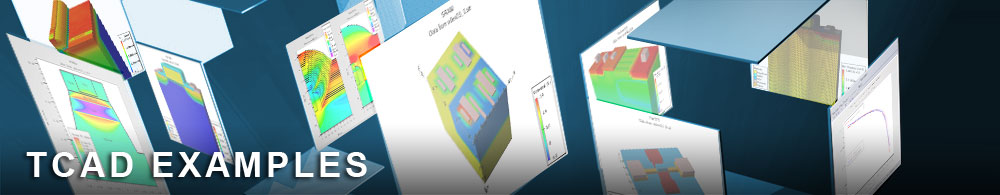PHEMT High Frequency Analysis

## hemtex06.in : PHEMT High Frequency Analysis

Requires: DevEdit/Blaze
Minimum Versions: Atlas 5.28.1.R

• AC parameters as a function of the gate bias
• Frequency domain AC calculation
• Calculation of s-parameters

This example is based on the previous example (see that text for details on the structure).

Once the structure is loaded into BLAZE, a small signal AC perturbation is applied at a frequency of 1 MHz to calculate the AC parameters (conductances and capacitances) as functions of Vgs. This also allows the user to obtain the cutoff frequency using the low frequency approximation using TonyPlot or using the extraction feature of DeckBuild. Currents, voltages, and AC parameters are saved in the log file, and internal structure information is saved in a solution file for the final bias condition of Vgs=0V, Vds=2 V.

The next step is a full frequency domain AC simulation when the Y parameter matrix (conductances and capacitances) are calculated as functions of frequency from 10 Hz up to 50 GHz. These results are stored in a log file. Additionally, with the specification of s.param on the log statement, the s-parameters are stored to the file.

At the end of simulation, parameter extraction statements are used to extract the following parameters using the extract feature of DeckBuild:

• Maximum gate-source capacitance
• Gate-source capacitance at Vgs=0
• Maximum cutoff frequency
• Cutoff frequency at Vgs=0
• Maximum AC current gain f=1MHz
• AC current gain at Vgs=0 f=1MHz

The results are also displayed using TonyPlot:

• Transconductance versus gate bias characteristic
• s-parameters: S12 & S21 in polar coordinates
• s-parameters: S11 & S22 on a Smith Chart

To load and run this example, select the Load button in DeckBuild > Examples. This will copy the input file and any support files to your current working directory. Select the Run button in DeckBuild to execute the example.